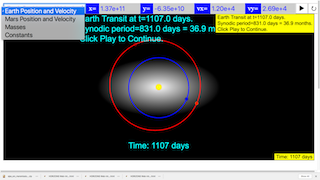Travelling to Mars

Traveling to Mars

Developed by A. Titus

Easy JavaScript Simulation version by Fremont Teng and Loo Kang Wee

In a contemporary, yet classic survival story, The Martian documents the struggle of astronaut Mark Watney to withstand the barrage of life-ending threats thrown by Mars. Although science fiction, author Andy Weir weaves physics, astronomy, botany, engineering, and chemistry into the book. One of the topics Weir deftly describes is the challenge imposed by traveling to Mars.

This set of activities focuses on the rocket’s orbit around the Sun, the “ideal” time to launch a rocket, and the initial conditions needed for the rocket to arrive at Mars. Students will determine the time between possible launches to Mars and the time required for the rocket to travel to Mars after launched.

 Subject Area Mechanics First Year and Beyond the First Year Glowscript and Easy JavaScript Simulation Students will be able to: obtain Cartesian coordinates for Earth and Mars (relative to the Sun) on a given date. (Exercise 1) use Newton’s Second Law to compute the orbits of Earth and Mars. (Exercise 1) demonstrate why NASA waits approximately 26 months between launches to Mars. (Exercise 1) use the Energy Principle to compute the speed of the rocket at a given distance from Earth. (Exercise 2) compute the orbit of the rocket using an initial velocity of the rocket when it is already well above Earth’s atmosphere. (Exercise 3) find initial conditions that takes a rocket to Mars and measure the time of travel for the rocket. (Exercises 3 - 4) 120 min
1instructorguide

There are two factors of Mars exploration explored by this activity:

1. The time between launches to Mars. (Relative positions of Earth and Mars is important.)
2. The time required to travel to Mars after “leaving” Earth.

The Euler-Cromer method can be used to compute the orbits. Thus, this activity is accessible to introductory physics students who have already modeled constant force motion. However, the activity can be extended for students in intermediate mechanics who might compute the motion of the rocket during launch.

Finding the day to launch and the initial direction to launch requires trial and error. It is possible to change parameters and re-run the simulation. However, it may be desirable to have an interface by which one can adjust the parameters and more easily rerun the simulation. This GlowScript simulation trip-to-Mars shows how you can use a second window to interactively set the initial velocity of the rocket using your mouse to adjust an arrow. Then, you can click a button on the day of launch. However, this kind of interactivity is not built into the attached template and sample code.

JPL’s Horizons web interface can be used to obtain initial velocity and position of Mars and Earth on today’s date. If you do not wish to obtain new data, you can use data obtained on Aug. 29, 2015.

variable value
${\stackrel{\to }{r}}_{Mars}$ $1000<-1.181709819034665E+08,2.135080519845017E+08,0>$ m
${\stackrel{\to }{v}}_{Mars}$ $1000<-2.028048448328753E+01,-9.673026032986073,0>$ m/s
${\stackrel{\to }{r}}_{Earth}$ $1000<1.371047699606275E+08,-6.350801741095807E+07,0>$ m
${\stackrel{\to }{v}}_{Earth}$ $1000<1.202828172495448E+01,2.690745455098789E+01,0>$ m/s

Exercise 1 leads students through the exercise of obtaining this data for whatever date they want.

In Exercise 3, a very important point to make to students is that our initial velocity of the rocket is specified relative to Earth. However, its velocity in the simulation must be relative to the Sun. Therefore, the initial velocity of the rocket when launched is

2Theory

According to Newton’s law of gravitation, the gravitational force by particle ${m}_{1}$ on particle ${m}_{2}$ is attractive and directed along a line between the masses with a magnitude

$\begin{array}{}\text{(2)}& {F}_{grav}=\frac{G{m}_{1}{m}_{2}}{{r}^{2}}.\end{array}$

In the first activity, you will determine the time that the relative position of Earth with respect to Mars repeats. This is called the synodic period of Earth and Mars. You will write a simulation of Earth and Mars and will find the time between Earth-Mars-Sun alignment (also called a Mars opposition.)

In the second activity, you will launch a rocket to Mars and measure the time required for the trip.

For a rocket launched to Mars, there are three gravitational forces on the rocket:

1. ${\stackrel{\to }{F}}_{\text{by Sun}}$
2. ${\stackrel{\to }{F}}_{\text{by Earth}}$
3. ${\stackrel{\to }{F}}_{\text{by Mars}}$

During the launch when the rocket is very close to Earth, the dominant forces on the rocket are thrust and the gravitational force by Earth. However we only want to study the gravitational forces on the rocket after it runs out of fuel and is orbiting the Sun. Therefore, we should start our simulation of the rocket when there is no thrust and the rocket is sufficiently far from Earth.

3Excercises

### EXERCISE 1

Write a simulation of Mars and Earth orbiting the Sun. You can neglect the interaction of Earth and Mars and only calculate the gravitational force by the Sun on each planet.

For the initial positions and velocities of the planets in your simulation, use JPL’s Horizons web interface. Here are the instructions for using Horizons.

1. Change the Ephemeris Type to “Vector Table” and click the “Use Selection Above” button to submit the change.

2. Change other settings too until you get the settings shown below (with the particular time span you are interested in).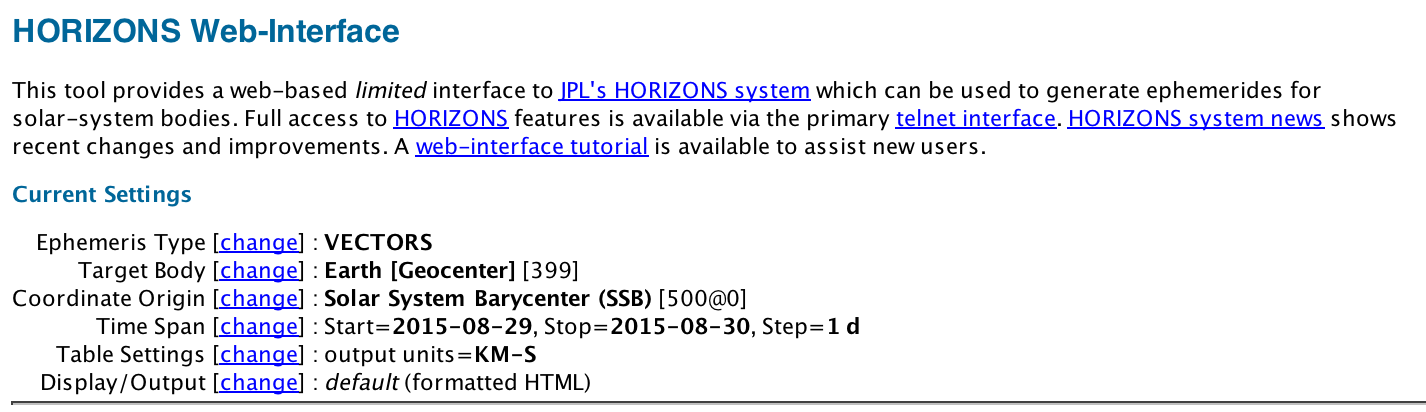1. Click the button “Generate Ephemeris.” Record the initial position vector and velocity vector in Cartesian coordinates for Mars. Note the units because you might use meters and seconds in your simulation.

2. Change the “Target Body” to Earth and repeat. Record the initial position vector and velocity vector in Cartesian coordinates for Earth. We will do a 2D simulation, so set the z-components of the position and velocity to zero.

Run your simulation and use it to determine the time (in days) between Mars oppositions, as shown below.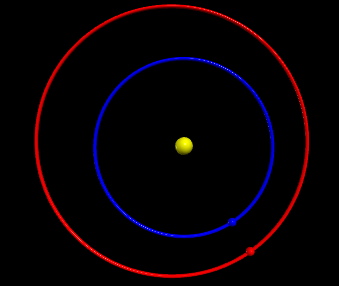In Exercise 2, you will find the best time (i.e. best relative positions of Earth and Mars) to launch the rocket from Earth. Do you think the best time (best positions of Earth and Mars) is when they are in opposition as shown above? After Exercise 3, you will be able to judge your answer.

### EXERCISE 2

You will eventually add a rocket to the simulation you wrote in Exercise 1, and you will find the direction of launch and day of launch that takes the rocket to Mars. However, we must first decide on the initial speed and position we should give the rocket.

To find the initial speed, we will assume:

• at some distance (of our choosing) from Earth, the boosters have burned out and we will call this the initial position of the rocket. Let’s choose an altitude equal to a geosynchronous orbit which is $4.6{R}_{E}$(a distance $5.6{R}_{E}$ from the center of Earth).
• near Earth, during the launch, the rocket must reach a speed greater than the escape speed. Earth’s escape speed is 11 km/s. To leave Earth and not return, the rocket will have to reach a speed of at least 11 km/s sometime after takeoff.
• our rocket had a speed of 11.5 km/s when it was near Earth’s surface and its final booster burned out; this was at such a high altitude that air resistance is negligible.

Using these assumptions and the Energy Principle, find the speed of the rocket when it is at an altitude of $4.6{R}_{E}$. What other assumptions did you make?

### EXERCISE 3

Depending on the particular orbit, it can take from 130 to 330 days to reach Mars. For NASA’s Mars missions, the average travel time is approximately 225 days, or about eight months. This is a long time, so you should definitely pack snacks and movies. The Mayflower took just over two months to cross the Atlantic. The journey to Mars is about four times longer than the Pilgrims’ journey from Europe to North America.

We will not model the rocket as its boosters are firing. Instead, we will set the initial velocity of the rocket as 5.4 km/s at a distance of 5.6 earth radii ($5.6{R}_{E}$) from the center of Earth. (This is an altitude of $4.6{R}_{E}$ from Earth.) Then we will try various launch days and launch directions for the rocket at this distance from Earth.

In writing your simulation, use the following assumptions:

1. The initial position of the rocket is $5.6{R}_{E}$ from the center of Earth. You can place the rocket at any point around Earth because the distance from Earth is small compared to the entire orbit of the rocket. Where on Earth it is launched should have a small effect.
2. The speed of the rocket at an altitude of 4.6 Earth radii is 5 km/s. You can set the direction to be any direction you choose. Let this be the rocket’s initial velocity. This speed is chosen because it ensures that the rocket was able to escape from Earth and is sufficiently far from Earth at the beginning of the simulation.
3. The thrusters are off during the entire flight. The net force on the rocket is the gravitational force by the Sun, Earth, and Mars.

Find a direction for the initial velocity of the rocket and find a day to launch (using the initial positions of the planets in Exercise 1) such that the rocket travels to Mars. Your program will have to compute orbits for the rocket, Earth, and Mars. You will have to try many different combinations of launch day and launch direction. Use trial and error, but learn from each trial to improve your guesses.

Also, think about what it means to “arrive at Mars.” Does it have to collide with Mars or merely get close enough that rocket boosters could conceivably change the orbit of the rocket?

### EXERCISE 4

Try different initial velocities and different days for the launch. Can you find more than one velocity and day that will give an orbit that will work?

5Solutions

### Exercise 1

Using initial positions on Aug 29, 2015, the first opposition occurs after 276 days.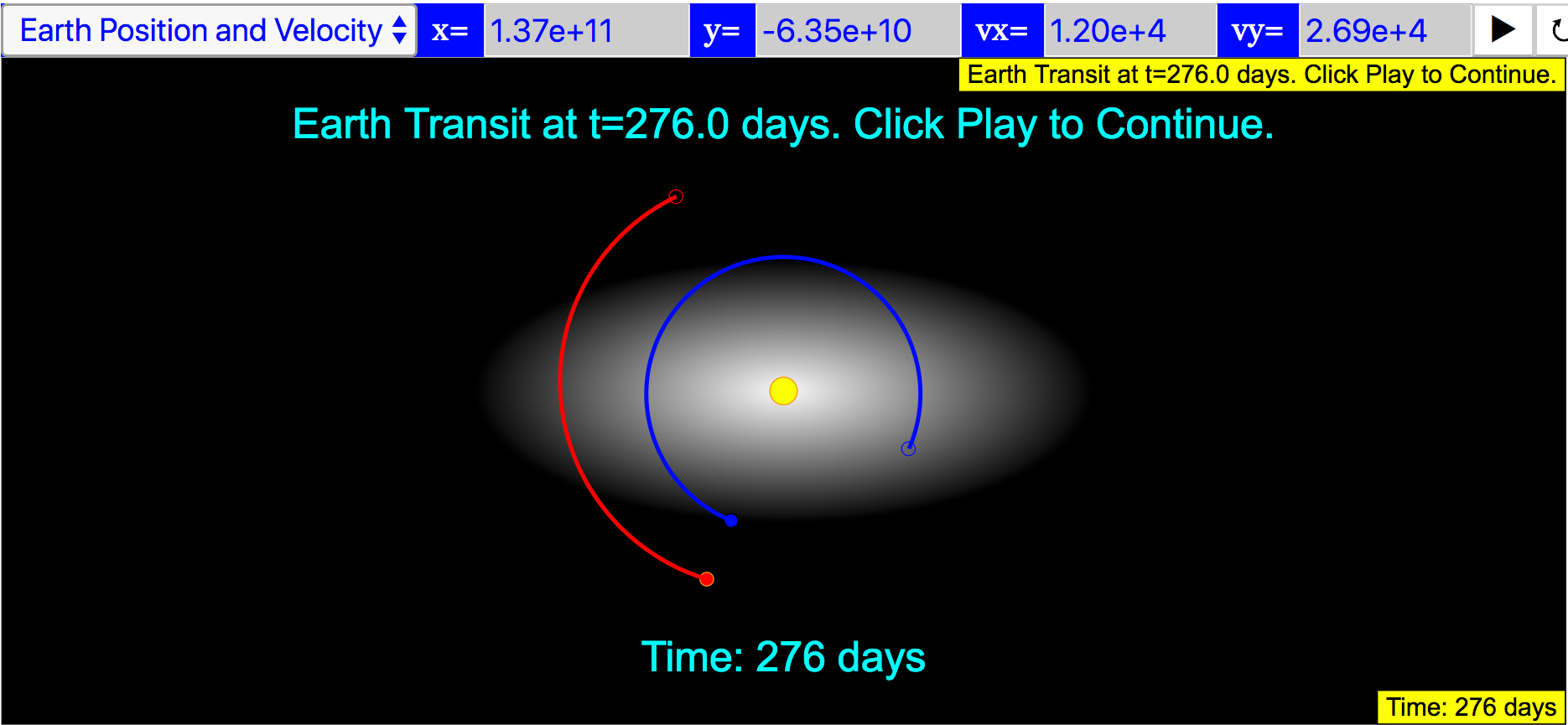The second opposition is at Day 1107.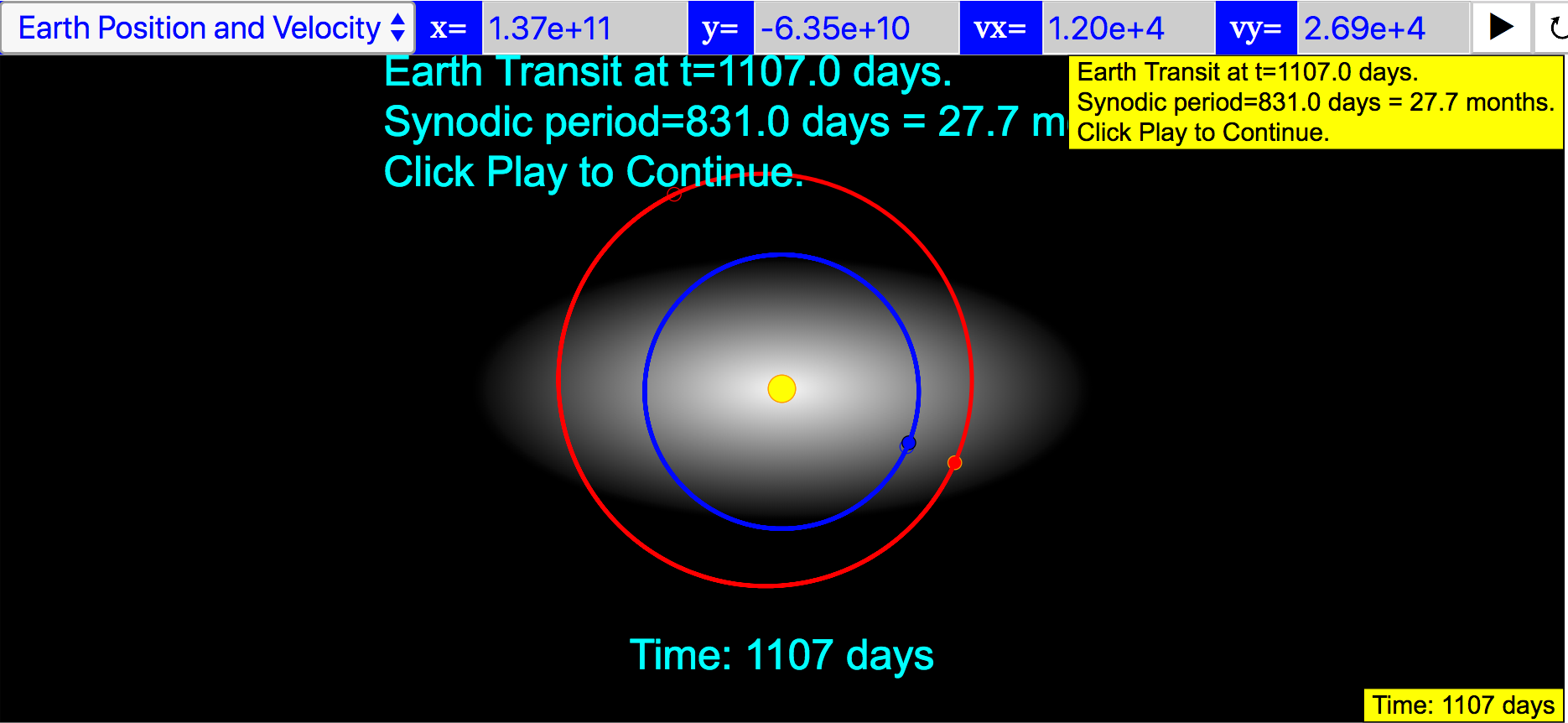The difference is 831 days, which is approximately 27.7 months.

### Exercise 2

The mass of Earth is $6×{10}^{24}$ kg. Its radius is $6.4×{10}^{6}$ m. The rocket is initially close to Earth so ${r}_{1}=\approx {R}_{E}$. Use the Energy Principle to find the speed of the rocket at ${r}_{2}=\approx 5.6{R}_{E}$. Define the system to be Earth and rocket. Assume there is no work or heat transferred to the system. Also, assume that the rocket’s interactions with the Sun and Mars are negligible during this change in altitude from Earth.

$\begin{array}{}\text{(9)}& {E}_{1}={E}_{2}\end{array}$
$\begin{array}{}\text{(10)}& {U}_{grav,1}+{K}_{1}={U}_{grav,2}+{K}_{2}\end{array}$
$\begin{array}{}\text{(11)}& \frac{-GmM}{{r}_{1}}+\frac{1}{2}m{v}_{1}^{2}=\frac{-GmM}{{r}_{2}}+\frac{1}{2}m{v}_{2}^{2}\end{array}$
$\begin{array}{}\text{(12)}& \frac{-2GM}{{r}_{1}}+{v}_{1}^{2}=\frac{-2GM}{{r}_{2}}+{v}_{2}^{2}\end{array}$
$\begin{array}{}\text{(13)}& {v}_{2}^{2}=\frac{2GM}{{r}_{2}}+\frac{-2GM}{{r}_{1}}+{v}_{1}^{2}\end{array}$
$\begin{array}{}\text{(14)}& {v}_{2}^{2}=2GM\left(\frac{1}{{r}_{2}}-\frac{1}{{r}_{1}}\right)+{v}_{1}^{2}\end{array}$
$\begin{array}{}\text{(15)}& {v}_{2}=\sqrt{2GM\left(\frac{1}{{r}_{2}}-\frac{1}{{r}_{1}}\right)+{v}_{1}^{2}}\end{array}$
$\begin{array}{}\text{(16)}& {v}_{2}\approx 5400\phantom{\rule{1em}{0ex}}\mathrm{m}/\mathrm{s}\end{array}$

### Exercise 3

The completed code uses initial positions on Aug 29, 2015. In this simulation, a possible launch is at Day 125, which is Jan. 01, 2016. Here is the starting point of the simulation.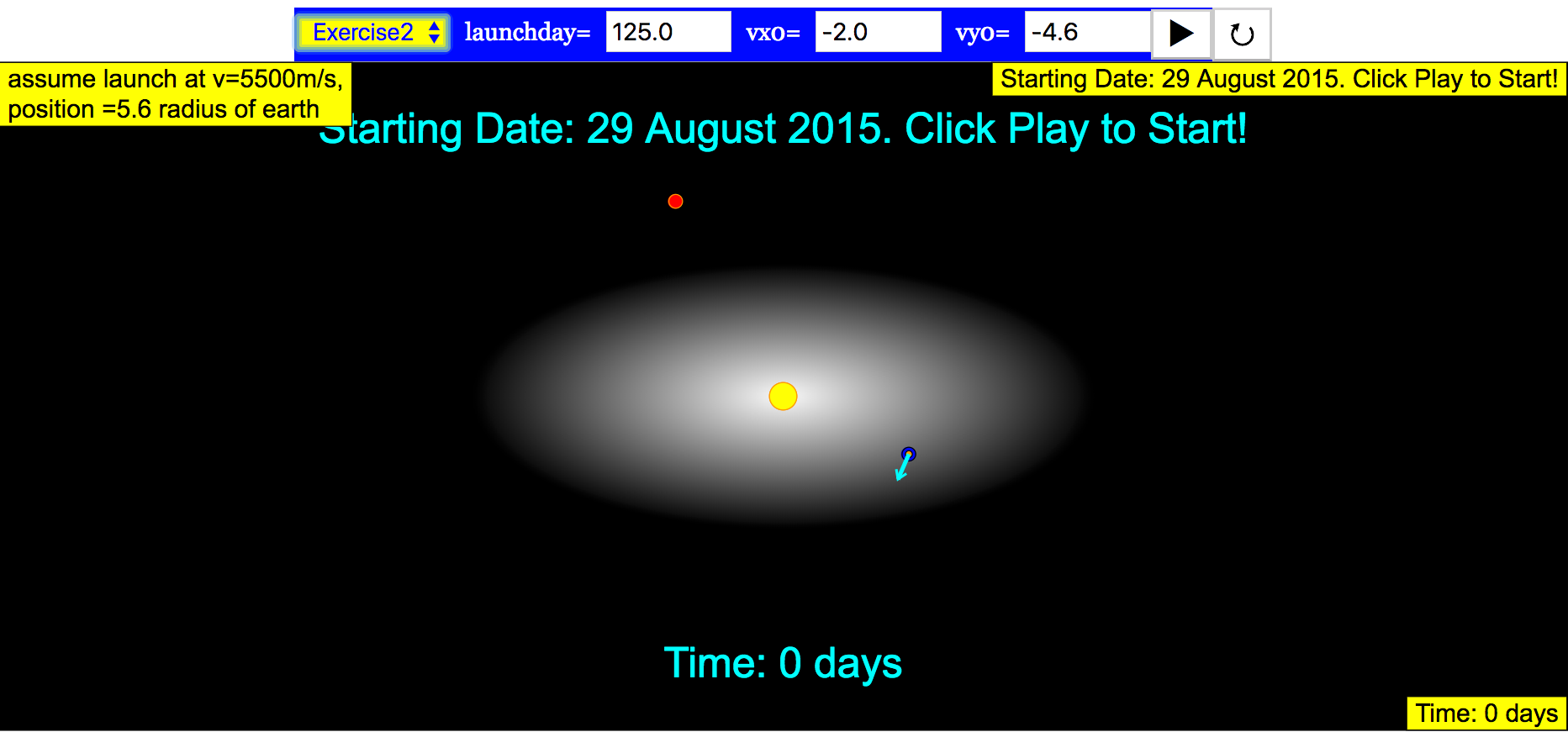The rocket arrives on June 25, 2016. This gives a travel time of 176 days which is approximately 6 months. The arrival is shown below (although “arrival” is defined as being within 200 Mars diameters.)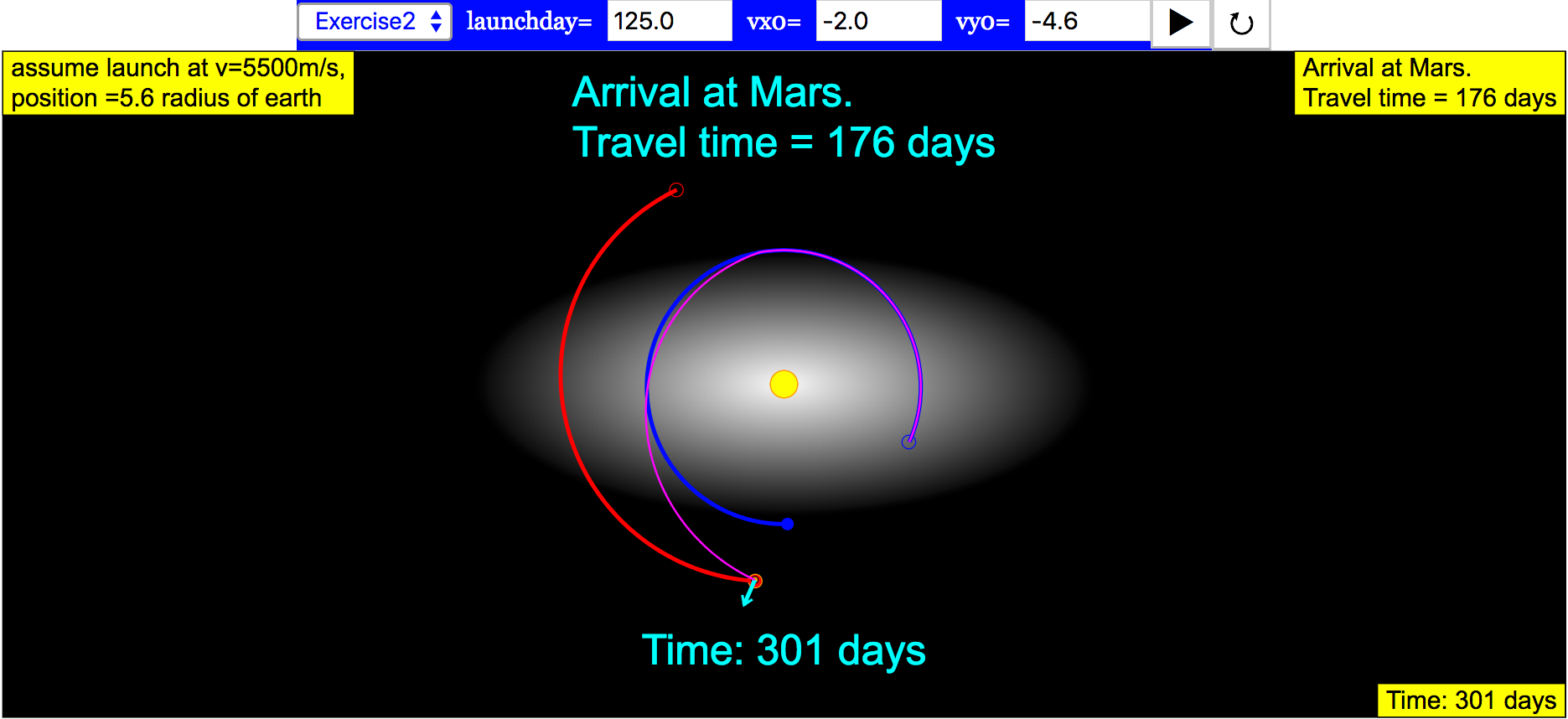It is worth comparing this result to actual missions. Space.com has an article that gives the following travel time for Mars missions.

Mission Travel Time
Mariner 4, the first spacecraft to go to Mars (1964 flyby) 228 days
Mariner 6 (1969 flyby) 155 days
Mariner 7 (1969 flyby) 128 days
Mariner 9, the first spacecraft to orbit Mars (1971) 168 days
Viking 1, the first U.S. craft to land on Mars (1975) 304 days
Viking 2 Orbiter/Lander (1975) 333 days
Mars Global Surveyor (1996) 308 days
Mars Pathfinder (1996) 212 days
Mars Odyssey (2001) 200 days
Mars Express Orbiter (2003) 201 days
Mars Reconnaissance Orbiter (2005) 210 days
Mars Science Laboratory (2011) 254 days

There are many solutions to this problem and it’s hard! Defining a large region of success for “reaching” Mars (like a radius of 500 Mars Diameters) is helpful.

### Exercise 4

It is best to collect data from the class on all of their successful orbits.

Glowscript with bug fixed Glowscript with bug fixed Rocket

GlowScript 2.7 VPython

#Developed by A.Titus

launchday=125 #day to launch

rinitial=5.6*6.4e6 #initial distance of rocket from center of earth

marsrocketdist=500*6.8e6 #distance from mars considered a success

vinitial=5.4e3 #initial speed of rocket

# v = vinitial*norm(vec(-1.7,-4.6, 0)) strangely EJSS values does not coincident with glowscript, need to use -1.4 instead of -1.7

v = vinitial*norm(vec(-1.4,-4.6, 0)) #initial velocity of rocket; change the direction only

#pos unit is m

#time unit is s

#pos and vel generated by http://ssd.jpl.nasa.gov/horizons.cgi

#Aug 29, 2015

#

#no z components

marspos=1000*vec(-1.176389005286295E+08,2.135936464902488E+08,0)

marsvel=1000*vec(-2.027713823922661E+01,-9.661960006152405E+00,0)

earthpos=1000*vec(1.376368513677354E+08,-6.342242292720988E+07,0)

earthvel=1000*vec(1.203162796791987E+01,2.691852057718956E+01,0)

#constants

AU=1000*149597870.7 #AU in m

G=6.67384e-11

#diameters used for drawing sun, mars, and earth; diameters are not to scale

Dsun=0.2*AU

Dearth=0.1*AU

Dmars=0.1*AU

#mass

Msun=1.989e30

Mearth=5.97219e24

Mmars=6.4185e23

Mrocket=1e4

#time

day=24*3600

dt=2*3600

t=0

#set up 3D scene

scene = display(width=430, height=400, userspin=False, userzoom=True)

scene.append_to_title("""<br>Click the simulation to begin.""")

scene.append_to_title("""<br><br>Assumptions include:""")

scene.append_to_title("""<br>1. The rocket's speed near Earth's surface is 12 km/s, relative to Earth.""")

scene.append_to_title("""<br>2. The velocity of the rocket at an altitude of 2 earth radii is 5.4 km/s in the direction shown by the arrow.""")

scene.append_to_title("""<br>3. The thrusters are off during the entire flight. The net force on the rocket is the gravitational force by the Sun, Earth, and Mars.<br><br>""")

#scales for arrows

scale1=Dsun

# set up 3D objects

sun=sphere(dispay=scene, pos=vec(0,0,0), radius=Dsun/2, color=color.yellow)

mars=sphere(dispay=scene, pos=marspos, radius=Dmars/2, color=color.red)

earth=sphere(dispay=scene, pos=earthpos, radius=Dearth/2, color=color.blue)

rocketarrow1=arrow(dispay=scene, pos=earth.pos, axis=scale1*norm(v), color=color.white)

# create trails

marstrail=attach_trail(mars, radius=0.2*Dmars, trail_type="points", interval=2, retain=1000)

earthtrail=attach_trail(earth, radius=0.2*Dearth, trail_type="points", interval=2, retain=1000)

rockettrail.stop

#create strings and labels

tstr="Time: {:.0f} days".format(0)

tlabel=label(pos=vector(0,1.2*mag(marspos),0), text=tstr)

launchstr="Starting Date: Aug. 29, 2015. \n"+launchday+" days until launch. \n Click to Run."

launchlabel=label(pos=vector(0,-1.2*mag(marspos),0), text=launchstr)

#set the range

scene.range=1.5*mag(marspos)

#this function is called when the rocket is launched

# it sets booleans and sets the initial velocitiy and momentum of the rocket

def launchRocket():

global vrocket, procket, rocketLaunched, justNowLaunched

rocketLaunched=True

justNowLaunched=True

vrocket=vearth+v

procket=Mrocket*vrocket

# initial positions, velocities, and momenta of all objects

earth.pos=earthpos

rocket.pos=earthpos

mars.pos=marspos

vearth=earthvel

vrocket=earthvel+v

vmars=marsvel

pearth=Mearth*vearth

pmars=Mmars*vmars

procket=Mrocket*vrocket

#booleans

rocketLaunched=False

run = False

justNowLaunched=False

# pause and then change the message

scene.waitfor('click')

launchstr="Launch Day "+launchday

launchlabel.text=launchstr

while True:

rate(200)

#earth

r=earth.pos-sun.pos

rmag=mag(r)

runit=norm(r)

Fearth=-G*Msun*Mearth/rmag**2*runit

pearth=pearth+Fearth*dt

vearth=pearth/Mearth

earth.pos=earth.pos+pearth/Mearth*dt

#mars

r=mars.pos-sun.pos

rmag=mag(r)

runit=norm(r)

Fmars=-G*Msun*Mmars/rmag**2*runit

pmars=pmars+Fmars*dt

mars.pos=mars.pos+pmars/Mmars*dt

#rocket

# launched, then compute Fnet, procket, and rocket.pos

if(rocketLaunched):

rocketarrow1.visible=False

#F by earth

r=rocket.pos-earth.pos

rmag=mag(r)

runit=norm(r)

Frocket_earth=-G*Mrocket*Mearth/rmag**2*runit

#F by sun

r=rocket.pos-sun.pos

rmag=mag(r)

runit=norm(r)

Frocket_sun=-G*Mrocket*Msun/rmag**2*runit

#F by mars

r=rocket.pos-mars.pos

rmag=mag(r)

runit=norm(r)

Frocket_mars=-G*Mrocket*Mmars/rmag**2*runit

#Fnet

Fnet=Frocket_sun+Frocket_earth+Frocket_mars

procket=procket+Fnet*dt

rocket.pos=rocket.pos+procket/Mrocket*dt

if(justNowLaunched):

justNowLaunched=False

#if not launched, just make the rocket at earth's position

else:

rocket.pos=earth.pos

#rocketarrow

rocketarrow1.pos=rocket.pos

#update time and label

t=t+dt

tstr="Time: {:.0f} days".format(t/day)

tlabel.text=tstr

#launch rocket on the launch day

if(t/day>launchday and rocketLaunched==False):

launchRocket()

#arrival at Mars

if(mag(rocket.pos-mars.pos)<marsrocketdist):

launchstr="Arrival at Mars. \nTravel time = {:.0f} days".format(t/day-launchday)

launchlabel.text=launchstr

scene.waitfor('click')

### Translations

Code Language Translator Run### Software Requirements

SoftwareRequirements

 Android iOS Windows MacOS with best with Chrome Chrome Chrome Chrome support full-screen? Yes. Chrome/Opera No. Firefox/ Samsung Internet Not yet Yes Yes cannot work on some mobile browser that don't understand JavaScript such as..... cannot work on Internet Explorer 9 and below

### Credits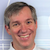Fremont Teng; Arron Titus; Loo Kang Wee

[text]

## Travelling to Mars JavaScript Simulation Applet HTML5

### Instructions

#### Control Panel

Toggling between the combo box will set their respective functions.

Selecting the first four options will give you fieldboxes to adjust their variables respective.

Selecting Exercise 1 will show the cproduct sign

Selecting the other Exercises will show you the Launch Rocket Settings

#### Play/Pause and Reset Buttons

Plays/Pauses and Resets the simulation respectively.
Note that you can also pause/play the simulation via double-clicking on the screen.

Research

[text]

[text]

### end faq

Rating 0.00 (0 Votes)